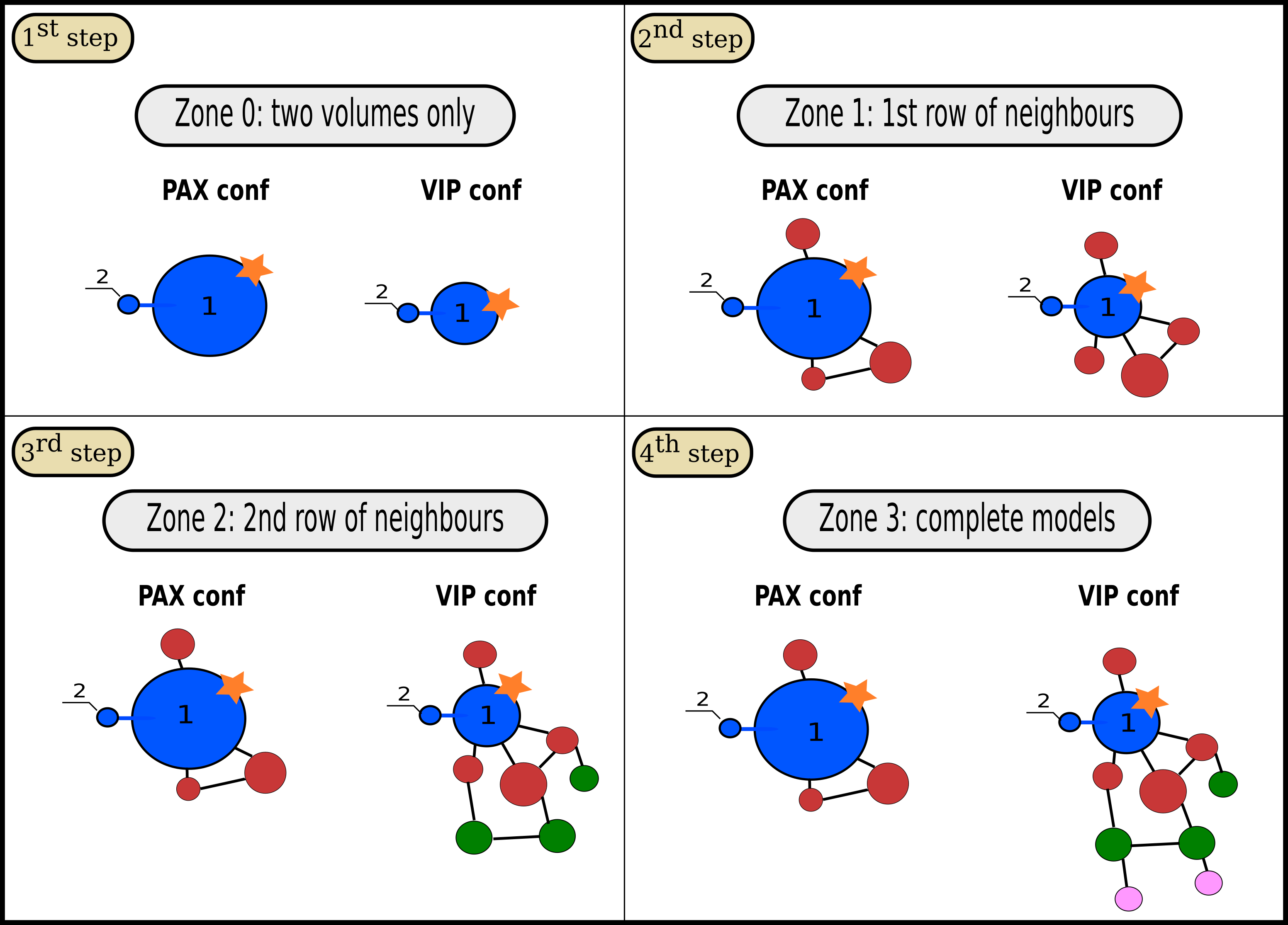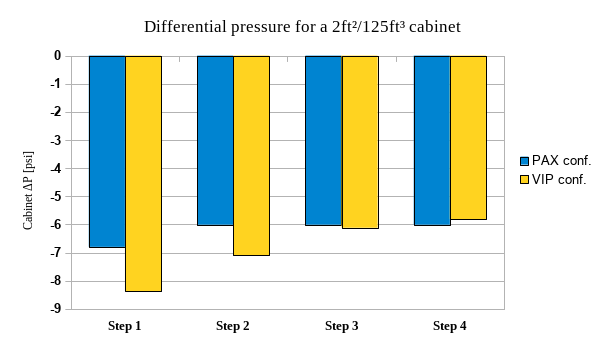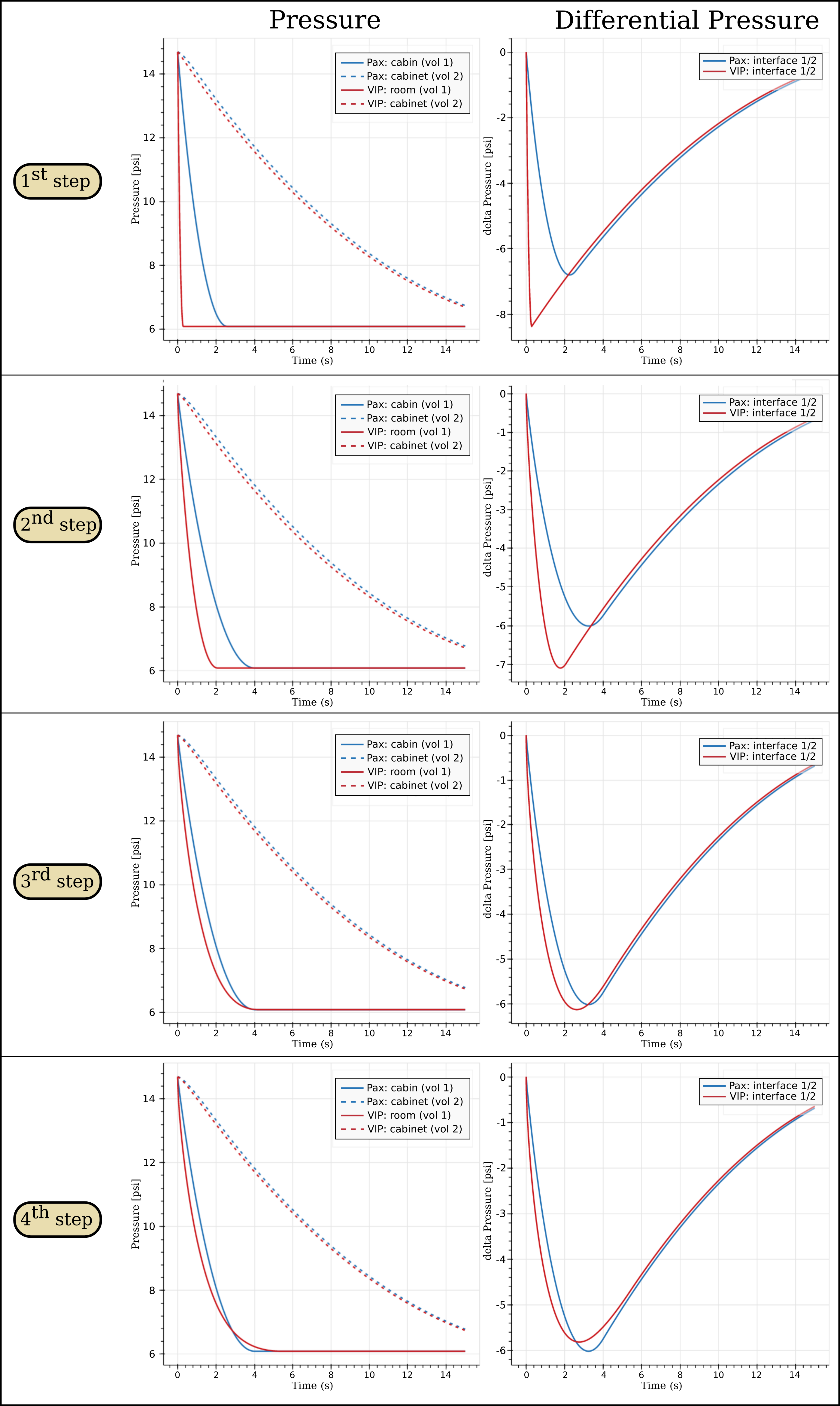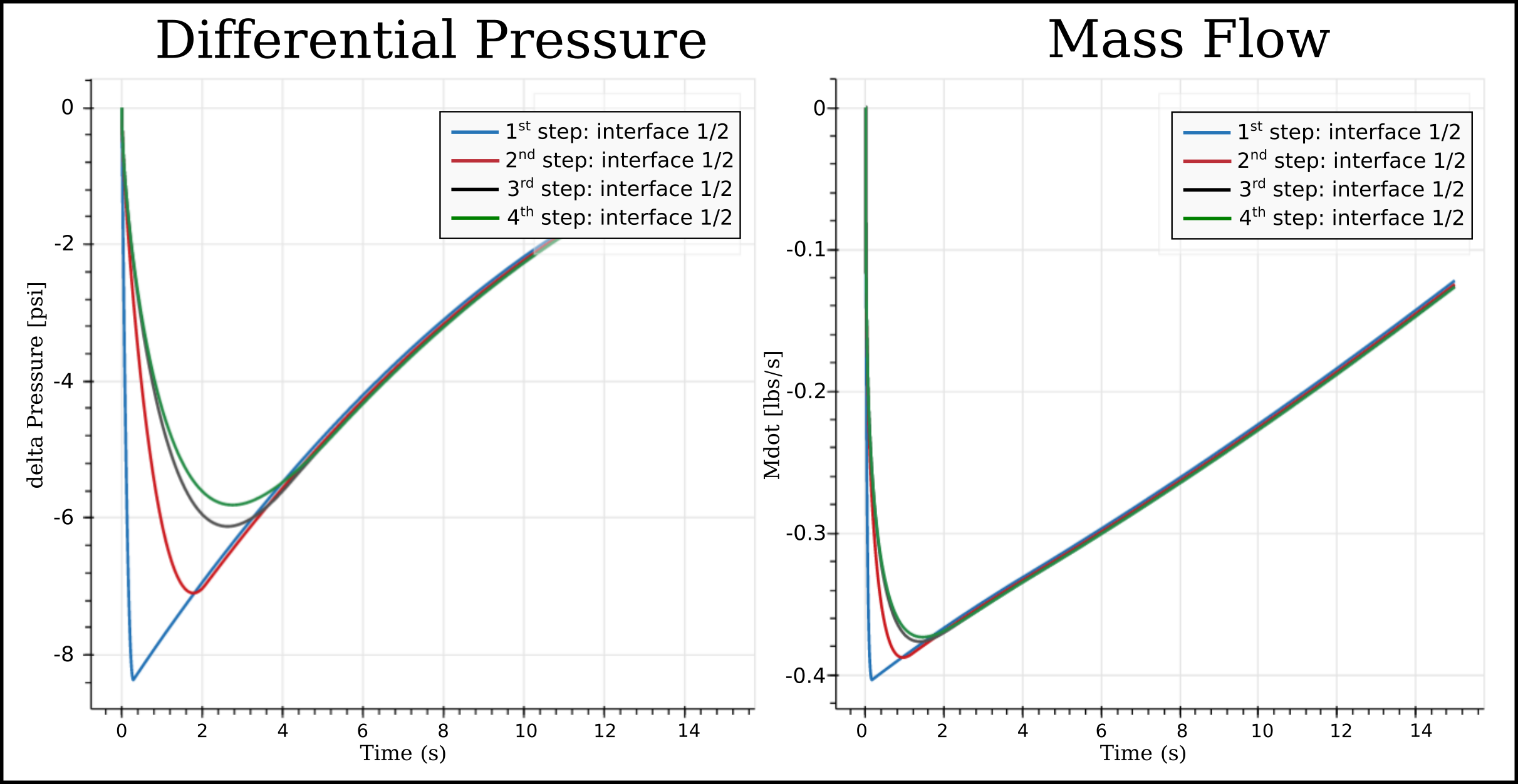# Small Volumes Decompression #6 - Airline X VIP Results Comparison (Further Step)

Published: jeu 20 décembre 2018
Updated: mar 29 janvier 2019

# Introduction

As shown in the Table of Article #5, in some cases the results difference ($\Delta_P {VIP} - \Delta_P {PAX}$) leads to negative values, see those cases highlighted below. It means that for our example - numeric Corporate Jet - those cases (big volume of Cabinets with tiny vent area), the $\Delta_P$ of the PAX configuration is higher than $\Delta_P$ of the VIP configuration.Generally one can expect that a same Cabinet installed in VIP configuration (enclosed in smaller room) will be exposed to higher $\Delta_P$ than when installed in PAX configuration (enclosed in bigger room - Main Deck). HOWEVER the negative values highlighted above proves that the the size of the room where the cabinet is enclosed is not the only factor to be considered.

To better understand this phenomenon, we selected one Cabinet Configuration (Cabinet Volume = 125ft³ and Vent Area = 2in²) to study the decompression process in detail, considering both configurations (PAX and VIP) of our dummy Numeric Corporate Jet. This Cabinet sustains the following maximum $\Delta_P$:

• 6.01psi - when installed in PAX configuration, Article #3.
• 5.81psi - when installed in VIP configuration, Article #4.

.

# Detailed Analysis of Cabinet (V=125ft³, VA=2in²)

In order to explain this surprising reduction of differential pressure in the VIP configuration a detailed analysis was performed in four steps as listed below:

• First step (Zone 0): a reduced model was considered with only 2 volumes: the Cabinet (vol 2) connected to the room where it is installed (vol 1).
• Second step (Zone 1): one additional row of neighbouring volumes is added to the PAX and VIP configuration models.
• Third step (Zone 2): one additional row of neighbouring volumes is added to the VIP configuration model.
• Fourth step (Zone 3): one additional row of neighbouring volumes is added to the VIP configuration model.NOTE: At the Second Step, the PAX configuration already includes all volumes whereas for the VIP configuration it only happens in the Fourth Step.

At each step a Sensitivity analysis (one key feature of Esonix) was considered to facilitate the comparison between PAX and VIP results.

NOTE: Additional information about Sensitivity analysis is available in the Esonix Manuals (see Manuals). Esonix Tutorials provides a good opportunity to practice the use of this feature (see Tutorials).

## Results

The chart and graphs below show the maximum $\Delta_P$ (psi) sustained by the Cabinet (Volume = 125ft³ and Vent Area = 2in²) in each one of the steps described above.In the following graphs the evolution of differential pressure seen by the cabinet is shown in the PAX and VIP configuration at each step previously defined.Based on chart and graphs above we can highlight some points:

• First Step: simplified models with two volumes (Cabinet and its hosting room), the size of the room is the only factor to be considered. As expected, the Cabinet installation in the VIP configuration (smaller room) is under higher $\Delta_P$ than in PAX configuration. The decompression event is much faster in the VIP configuration (smaller room volume) than in PAX configuration (bigger room volume), as shown by the graph above.

• Second Step: when one row of neighbouring volumes is added in both configurations, new factors, besides the size of the room, were added to the analysis. We can observe that the decompression event spends more time to achieve the maximum $\Delta_P$, which decreases in both configurations, see graph above.

• Third Step: a new row of neighbouring volumes is added in the VIP configuration. We can also observe that the decompression event spends still more time to achieve the maximum $\Delta_P$. The maximum $\Delta_P$ decreases and approaches the maximum $\Delta_P$ from PAX configuration, already stable.

• Fourth Step: the last row of neighbouring volumes is added to the VIP configuration. At this point both configurations are “complete”. We can observe that the new row of neighbouring volumes brings even more complexity to the model, increasing the time of the decompression event and decreasing the maximum $\Delta_P$. We can also observe that at this point the maximum $\Delta_P$ of the PAX configuration becomes higher than the maximum $\Delta_P$ of the VIP configuration.

In other words we can say that starting from a simple model (two volumes), each time an additional row of neighbouring volumes is considered, it brings additional complexity to the model. Some of the complexities can be exemplified as Air flow change and increase of the time to achieve the maximum $\Delta_P$.

The graphs below compare the $\Delta_P$ curves and Mass Flow (Mdot) of the Connection #1 (between Cabinet and room) of all models representing the VIP configuration in each step of the detailed analysis. By those graphs one can easily observe that at each step, when a new row of neighbouring volumes is added, the maximum $\Delta_P$ decreases, the decompression spend more time to achieve the stability and the Mass Flow becomes slower.# Conclusion

In this article we have taken a step further in the study of Small Volume Decompression demonstrating that to determine the actual $\Delta_P$ sustained by an enclosed Cabinet several factors should be evaluated.

We can conclude, for example, that the maximum $\Delta_P$ sustained by an enclosed Cabinet depends not only of the size of the room where it is installed but also of the complexity of the design and how the volumes are connected between them.

Esonix is an easier and efficient tool to investigate the actual pressure sustained by a Cabinet in case of decompression. With Esonix software and its features, the user is able to check several models at the same time and perform comparisons between them.

## NEXTSTEP…

In the next Article we will study a possible approach to decrease the pressure sustained by an enclosed Cabinet in case of aircraft decompression.

See here the list of Articles referring to the study of Small Volume Decompression:

• Article #1: Small Volumes Decompression #1 - Understanding the Problem

• Article #2: Small Volumes Decompression #2 - Airline/Pax, VIP configurations and Aircraft Parameters

• Article #3: Small Volumes Decompression #3 - Analysis of closed compartment in the Cabin Airline/PAX Configuration

• Article #4: Small Volumes Decompression #4 - Analysis of closed compartment in the Cabin VIP Configuration

• Article #5: Small Volumes Decompression #5 - Airline X VIP Results Comparison

• Article #6: Small Volumes Decompression #6 - Airline X VIP Results Comparison (Further Step)

• Article #7: Small Volumes Decompression #7 - Decompression Analysis considering the Cabinet Stiffness

• Article #8: Small Volumes Decompression #8 - Study summary and conclusions